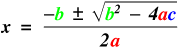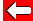# Quadratic Equation Formula

Life gets much more complicated when we have to solve equations of the form ax² + bx + c = 0. Fortunately there's a formula we can use to solve them:Let's use this equation to solve: x² - x - 2 = 0

To solve, all we need to know are the values of a, b and c from the standard equation. In this case 1, -1 and -2 respectively.

N.B. This formula is normally given on exam papers - phew!Go back a pageMaths MenuGo to next page